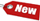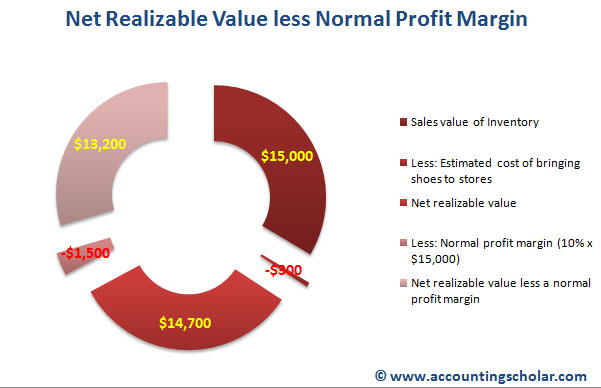Browse Accounting Lessons HereAccounting Terms & DefinitionsAccounting for Merchandising ActivitiesDebits and Credits (Double Entry Accounting)Business Valuation FormulasTime Value of Money & Present/Future ValuesComplex Debt & Equity InstrumentsCommon Stock & Shareholder's EquityAccounting & Finance RatiosValuing Common StockCorporate Income TaxesLower of Cost or Market (LCM) & Inventory ValuationChart of Accounts & BookkeepingBonds Payable & Long Term LiabilitiesCapital AssetsGAAP, Accrual & Cash Accounting, Information Commodity, Internal Controls & MaterialityWhat category of browser are you on this website? Accounting student (homework help) Finance professor (university research) Accounting manager (at work) Other Explore Careers in Accounting and FinanceVisit our section on Careers in Accounting & Finance to explore vast opportunities in this industry.

Chapter 3.2® - Example of Net Realizable Value less a Normal Profit Margin, Market equals = Net Realizable value or Market equals net realizable value minus a normal Profit Margin

Let’s consider an example where we lower the carrying value of inventory using the net realizable value less a normal profit margin method. Consider a sporting goods company owns shoes worth \$13,500 at December 31st, 2009; these shoes have a selling value of \$15,000. The estimated cost of bringing these shoes to its stores for resale is \$300 and a normal profit margin is 20%. How do we determine “market” in such a case?

 Sales value of Inventory \$15,000 Less: Estimated cost of bringing shoes to stores (\$300) Net Realizable Value \$14,700 Less: Normal profit margin (10% x \$15,000) (\$1,500) Net realizable value less a normal profit margin \$13,200This pie graph shows a break down of the sales value of inventory less estimated costs of bringing the shoe inventory to stores & the net realizable value. A normal profit margin is deducted of the net realizable value to arrive at 'net realizable value less a normal profit margin.'

Let's consider 2 scenarios where in i) Market equals Net Realizable value and ii) Market equals net realizable value minus a normal profit margin.

i) Market equals = Net Realizable value

If market equals the net realizable value alone, then we see that with an original purchasing cost of \$13,500, there will be a \$1,200 gain reported on the income statement, in the form of reduced cost of goods sold (\$13,500 - \$14,700) because instead of reporting Cost of Goods Sold of \$14,700 which is the market, we will recognize the original purchasing cost of \$13,500 as COGS and deduct this from Net Sales. In 2011, when we record \$13,500 as the cost of inventory (due to lower of cost or market with cost being lower at \$13,500), we will realize a gain of \$1,200 on the shoes inventory after deducting the cost of resale of \$300.

 Inventory Value December 31st, 2010 Balance Sheet Profit / Loss Recognition on the Income Statement a) If Market = NRV In 2010 In 2011 Cost = \$13,500 Market = \$14,700 LCM = \$13,500 \$13,500 Effect on 2010 Income Statement (\$13,500 - \$14,700) \$1,200 Profit Effect on 2011 Income Statement (when Inventory is sold) Revenue ----> \$15,000 Cost of Inventory > (\$13,500) Cost of Resale ---> (\$300) Profit --------> \$1,200

ii) Market equals = Net Realizable Value minus a Normal Profit Margin

In the second example where market equals the net realizable value minus a profit margin, there will be a \$300 loss reported on the income statement in 2010 because we are using the lower of cost (\$13,500) or market (\$13,200), thus we are using the market. Notice however that we benefit in 2011 because when subtracting this \$13,200 market from revenue, we derive a profit of \$1,500. If we had used the higher cost of \$13,500, we would be short \$300 profit because the calculations would look like:

 Inventory Value December 31st, 2010 Balance Sheet Profit / Loss Recognition on the Income Statement b) If Market = NRV minus a normal profit margin In 2010 In 2011 Cost = \$13,500 Market = \$13,200 LCM = \$13,200 \$13,200 Effect on 2010 Income Statement (\$13,500 - \$13,200) \$300 Loss Effect on 2011 Income Statement (when Inventory is sold) Revenue ----> \$15,000 Cost of Inventory > (\$13,200) Cost of Resale ---> (\$300) Profit --------> \$1,500